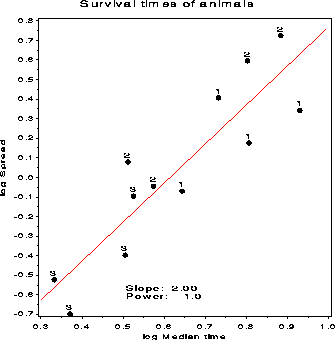sprdplot Find power transformations to equalize variance sprdplot

# SAS Macro Programs: sprdplot

\$Version: 1.2-1 (27 Feb 2013)
Michael Friendly
York University

## The sprdplot macro (get sprdplot.sas)

### Find power transformations to equalize variance

The sprdplot macro produces a spread-level plot to determine if a simple power transformation can equalize within-group variance of a response variable in a dataset classified by one or more classification variables.

The spread-level plot has the property that *if* the relationship between log10(Interquartile range) and log10(Median) is reasonably linear, then the recommended power is p = 1 - slope, and the transformation is

```           / y**p,      p > 0
y --> | log(p),    p = 0
\ -100y**p,  p < 0
```
The macro chooses the best power(s) from a list of simple integers and half-integers (PLIST=), and creates new variables using those transformations.

### Method

The power is determined from the slope of a weighted linear regression of log10(IQR) on log10(Median), using sample sizes as weights.

## Usage

The SPRDPLOT macro is defined with 11 keyword parameters. The VAR= and CLASS= parameters are required. The arguments may be listed within parentheses in any order, separated by commas. For example:
```  %sprdplot(data=animals, var=survive, class=treat poison);
```

### Parameters

Default values are shown after the name of each parameter.
DATA=
Name of the input dataset [Default: `DATA=_LAST_`]
CLASS=
[R] Names of one or more class variables. Only the first CLASS= variable is used as point labels in the graphics plot.
VAR=
[R] Name of the variable to be transformed. Must be numeric, and should contain all positive values.
OFFSET=
Constant added to the VAR= variable before transformation. If the variable contains negative values, OFFSET is set equal to the `abs(minimum)` value, to ensure that all values are positive.
PREFIX=
Prefix for name of transformed variable. If the PREFIX is T_ and `BEST=1`, the transformed variable is named T_&var. If BEST>1, the variables are named T_1&var, T_2&var, ... [Default: `PREFIX=T_`]
PLIST=
List of powers to consider. Should be a blank-separated list of numbers in increasing order. [Default: PLIST=-3 -2 -1 -.5 0 .5 1 2 3]
BEST=
Number of best powers to transform `&var` [Default: `BEST=1`]
PPLOT=
Produce a printer plot? [Default: `PPLOT=N`]
GPLOT=
Produce a graphics plot? [Default: `GPLOT=Y`]
HTEXT=
Height of text in graphics plot [Default: `HTEXT=1.7`]
OUT=
Name of the output dataset [Default: OUT=&DATA]

### Example

The data give survival times (in 10 hour units) of animals exposed to one of 3 types of poison and given one of 4 treatments, in a (3 x 4) design, with 4 replications. Box and Cox (1964) showed that a reciprocal transformation is reasonable.
```%include macros(sprdplot);        *-- or include in an autocall library;

title 'Survival times of animals';
* Hand etal #403, from Box & Cox;
data animals;
do poison=1 to 3;
do rep = 1 to 4;
do treatmt='A', 'B', 'C', 'D';
input time @;
time = time*10;
output;
end;
end;
end;
label treatmt='Treatment' time='Survival time (hrs)';
cards;
0.31  0.82  0.43  0.45  0.45  1.10  0.45  0.71
0.46  0.88  0.63  0.66  0.43  0.72  0.76  0.62
0.36  0.92  0.44  0.56  0.29  0.61  0.35  1.02
0.40  0.49  0.31  0.71  0.23  1.24  0.40  0.38
0.22  0.30  0.23  0.30  0.21  0.37  0.25  0.36
0.18  0.38  0.24  0.31  0.23  0.29  0.22  0.33
;
*-- Check for variance dependent on mean;
%sprdplot(data=animals, class=poison treatmt, var=time);
```
This produces the graph, chooses p= -1 (i.e., -100/Time), which is saved in the variable T_TIME.```*-- Analyze the transformed response (T_TIME = -1/Time);
proc glm data=animals;
class poison treatmt;
model t_time = poison | treatmt;
```

boxglm
meanplot
symplot